#Function Repository Resource:

# ReapAssociation

Equivalent to Reap, but returns an association with tags as keys

Contributed by: Richard Hennigan (Wolfram Research)
 ResourceFunction["ReapAssociation"][expr] gives the value of expr together with all expressions to which Sow has been applied during its evaluation. Expressions sown using Sow[e] or Sow[e,tagi] with different tags are returned as lists in an association keyed by the tags. ResourceFunction["ReapAssociation"][expr,patt] reaps only expressions sown with tags that match patt. ResourceFunction["ReapAssociation"][expr,{patt1,patt2,…}] puts expressions associated with each of the patti in a separate list. ResourceFunction["ReapAssociation"][expr,patt,f] has values f[tagi(ei,1,ei,2,...}] for each key tagi.

## Details and Options

Sow and ResourceFunction["ReapAssociation"] provide a convenient way to accumulate a list of intermediate results in a computation.
ResourceFunction["ReapAssociation"] accumulates expressions in the order in which Sow is applied to them.
Expressions sown with a particular tag are collected by the innermost ResourceFunction["ReapAssociation"] whose pattern matches the tag.
Expressions sown with no tag are returned using the tag None.
The result of evaluating the expression is returned using the key "Result", and the sown values are in an association under the key "Sown".
ResourceFunction["ReapAssociation"][expr] is equivalent to ResourceFunction["ReapAssociation"][expr,_].
ResourceFunction["ReapAssociation"] has attribute HoldFirst.

## Examples

### Basic Examples

Evaluate a sequence of expressions, "reaping" ones that have been "sown":

 In:=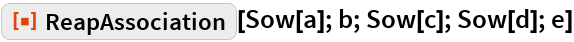Out=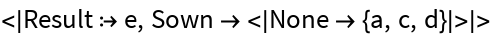Compute a sum, "reaping" i^2 "sown" at each step:

 In:=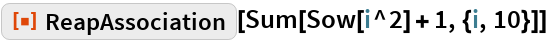Out=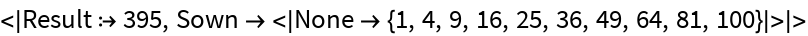Make a separate key for each tag being reaped:

 In:=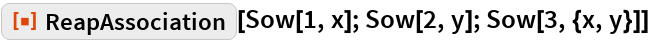Out=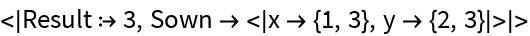In:=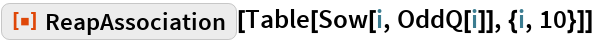Out=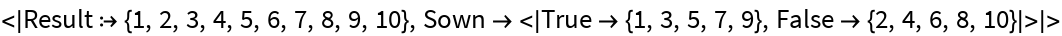In:=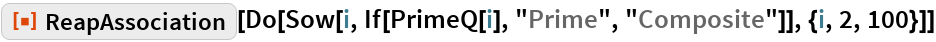Out=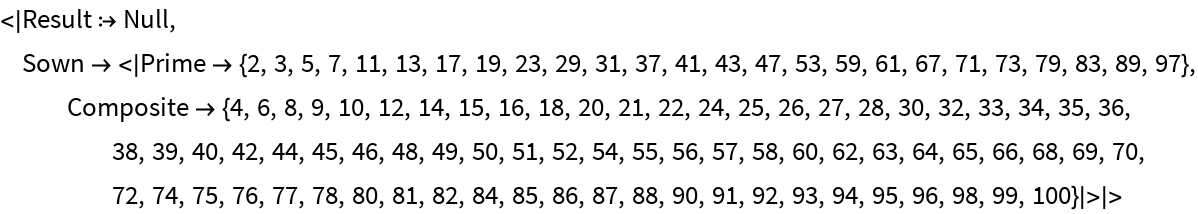### Scope

If nothing is sown, an empty association will be returned in the "Sown" key:

 In:=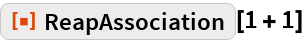Out=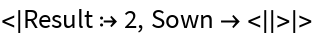Count the number of instances of each integer, separating the negative:

 In:=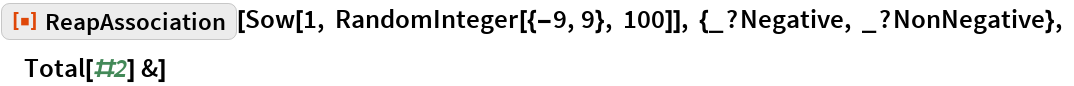Out=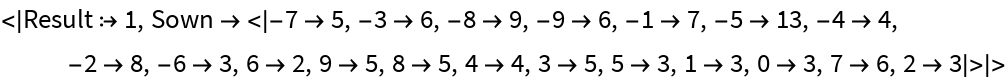### Generalizations & Extensions

Apply f to each distinct key and list of values:

 In:=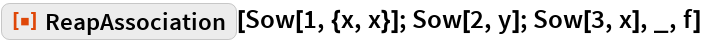Out=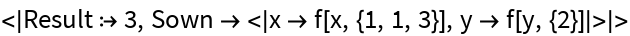In:=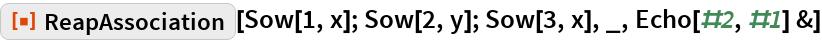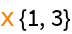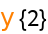Out=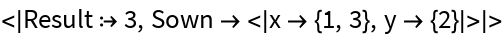### Applications

Find the list of values sampled by Plot:

 In:=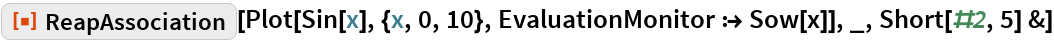Out=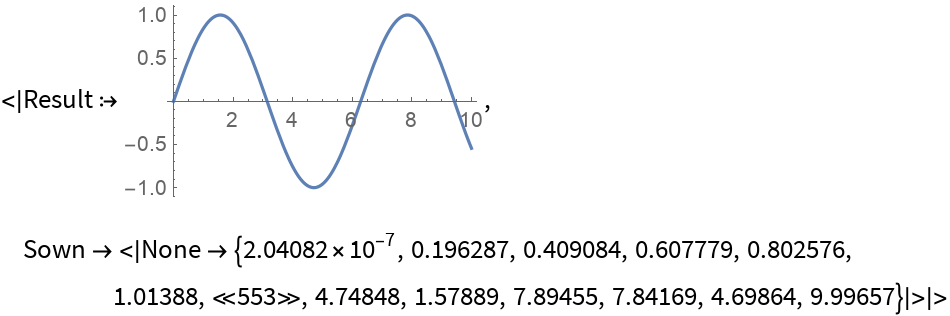Remove duplicates from a list, keeping the original order:

 In:=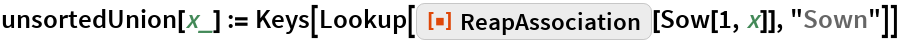In:=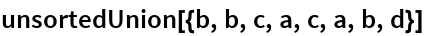Out=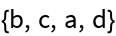This is like Union without the sorting:

 In:=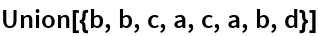Out=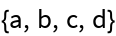### Properties & Relations

If no expressions are sown, ReapAssociation returns an empty association of expressions it has reaped:

 In:=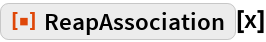Out=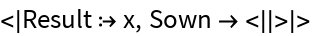ReapAssociation collects expressions in exactly the order they are sown:

 In:=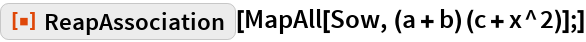Out=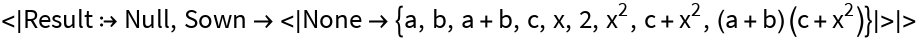The list associated with the first tag to be encountered will be the first key:

 In:=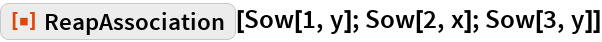Out=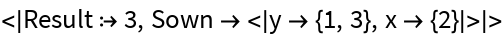When the order of tags is not known, ReapAssociation can make it easier to understand results compared to Reap:

 In:=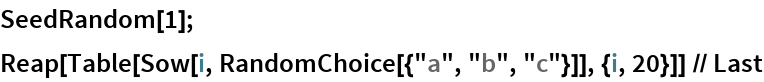Out=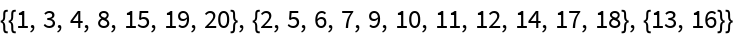In:=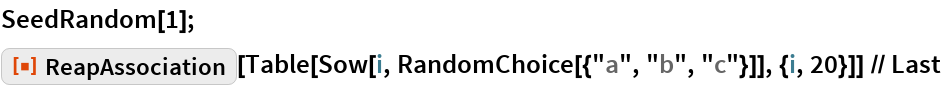Out=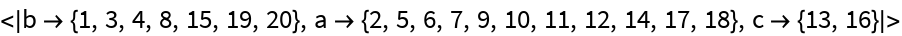### Neat Examples

Create an animation showing the full stack history of an evaluation:

 In:=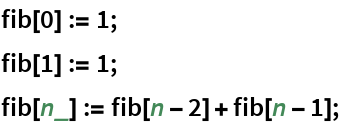In:=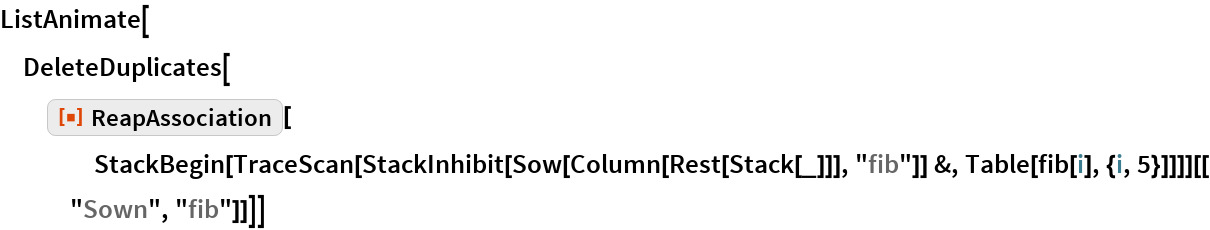Out=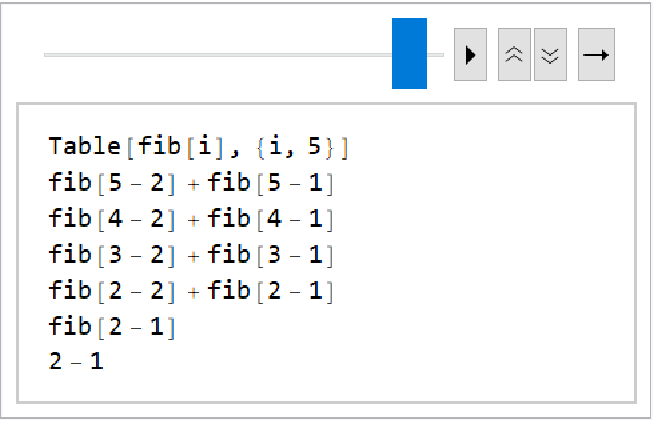## Requirements

Wolfram Language 11.3 (March 2018) or above